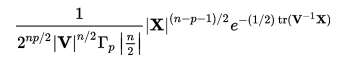# Wishart Distribution: Basic Definition, Uses

Share on

## What is the Wishart Distribution?

The Wishart distribution is one of the more complicated distributions in statistics, partly because there are many ways to define it. First of all, the simplest definition: it is the multivariate counterpart of a chi-squared distribution when the degrees of freedom are not integers. For integer degrees of freedom, it is the multivariate counterpart of the gamma distribution.

In inferential statistics, the Wishart distribution is also defined as the distribution of the sample covariance matrix. The covariance matrix is created from a random sample drawn from a multivariate normal distribution. It is usually denoted as Wp(Σ, n) where Σ is the covariance matrix and n is the number of degrees of freedom.

Kollo and Von Rosen (2005) define the Wishart in terms of a matrix distribution:

The matrix W : p x p is said to be a Wishart distribution if and only if W = XX’ for some matrix X, where X ~ Np,n(M,Σ,I), and Σ is positive definite. If M = 0, the distribution is a central Wishart (W ~ Wp(Σ,n)); if M ≠ 0, the distribution is non central (Wp(Σ,n,Δ)), where Δ = MM’)

.

## Uses

One of the Wishart Distribution’s main uses is to approximate a covariance matrix, as long as the underlying distribution is normal. In addition, many different sum of squares and product matrices also have Wishart distributions.

## PDF

The probability density function (PDF) for the Wishart distribution is complex and doesn’t exist unless the sample size is greater than the number of variables in the model (Abell et. al).

The formula for the PDF is:Where:

References:
Abell et. al. Statistics with Mathematica.
Kollo, T. and Von Rosen, D. (2005). Advanced Multivariate Statistics with Matrices. Springer, Dordrecht.

CITE THIS AS:
Stephanie Glen. "Wishart Distribution: Basic Definition, Uses" From StatisticsHowTo.com: Elementary Statistics for the rest of us! https://www.statisticshowto.com/wishart-distribution/
---------------------------------------------------------------------------Need help with a homework or test question? With Chegg Study, you can get step-by-step solutions to your questions from an expert in the field. Your first 30 minutes with a Chegg tutor is free!